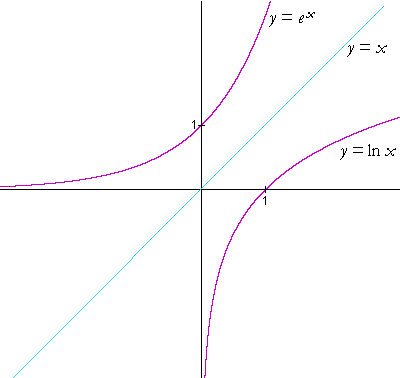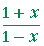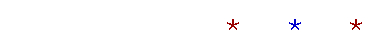Topics in

P R E C A L C U L U S

21

# LOGARITHMIC AND EXPONENTIAL FUNCTIONS

Exponential functions

THE LOGARITHMIC FUNCTION WITH BASE b is the function

y   =  logb x.

b is normally a number greater than 1 (although it need only be greater than 0 and not equal to 1).  The function is defined for all x > 0.  Here is its graph for any base b.Note the following:

•  For any base, the x-intercept is 1.  Why?

The logarithm of 1 is 0.  y = logb1 = 0.

Topic 20

•  The graph passes through the point (b, 1).   Why?

The logarithm of the base is 1.  logbb = 1.

•  The graph is below the x-axis—the logarithm is negative—for

0 < x < 1.

Which numbers are those that have negative logarithms?

Proper fractions.

Lesson 20 of Arithmetic

•   The function is defined only for positive values of x.

logb(−4), for example, makes no sense. Since b is always positive,

no power of b can produce a negative number.

•  The range of the function is all real numbers.

•  The negative y-axis is a vertical asymptote.

Example 1.  Translation.   Here is the graph of the natural logarithm,

y = ln x.And here is the graph of   y = ln (x − 2)—which is its translation 2 units to the right.The x-intercept has moved from 1 to 3.  And the vertical asymptote has moved from x = 0 to x = 2.

Problem 1.   Sketch the graph of  y = ln (x + 3).This is a translation 3 units to the left.   The x-intercept has moved from 1 to −2.  And the vertical asymptote has moved from x = 0 to x = −3.

## Exponential functions

By definition:

logby = x   means   bx = y.

Corresponding to every logarithm function with base b, we see that there is an exponential function with base b:

y   =  bx.

The argument of an exponential function is the exponent.

An exponential function is defined for every real number x.  Here is its graph for any base b:There are two important things to note:

•  The y-intercept is at (0, 1).  b0 = 1.

•  The negative x-axis is a horizontal asymptote.  For when x is a large negative number—e.g. b−10,000—then y is a very small positive number.

Problem 2.

a)   Let f(x) = ex.  Write the function f(−x).

f(−x)   =  ex

The argument x is replaced by −x.

Topic 3

b)   What is the relationship between the graph of  y = ex  and the graph
b)    of  y = ex ?

y = e−x is the reflection about the y-axis of y = ex.

Topic 15

c)   Sketch the graph of y = ex.Inverse relations

The exponential function and logarithmic function with base b are inverses.

f(x) = logbx  and  g(x) = bx  are inverses.

They satisfy the definition.

f(g(x))  =  f(bx) = logbbx = x.

We saw that in the previous Topic.

And:

g(f(x))  =  g(logbx) = blogbx) = x.

That embodies the definition of a logarithm:  logbx is the exponent to which b must be raised  to produce x.

Problem 3.   Evaluate the following.

 a)  log225 =  5 b)  log552x =  2x c)  log 106.2 = 6.2 d)  2log25 =  5 e)  5log5(x − 1) =  x − 1 f)  10log 100 =  100

Problem 4.

a)   What function is the inverse of  y = ln x?

y = ex.

b)   Let  f(x) = ln x   and  g(x) = ex,   and show that f and g satisfy the
b)   inverse relations.

f(g(x)) = ln ex = x,

g(f(x)) = eln x = x.

Here are the graphs of  y = ex   and   y = ln x :As with all pairs of inverse functions, their graphs are symmetrical with respect to the line  y = x.  (See Topic 19.)

Problem 5.   Evaluate the following.

 a)  ln ex + 1 =  x + 1 b)  eln (x − 5) = x − 5

Problem 6.   Evaluate  ln earccos (−1).

ln earccos (−1) = arccos (−1) = π.

"The angle whose cosine is −1 is π."

Topic 19 of Trigonometry.

Exponential and logarithmic equations

Example 2.   Solve this equation for x :

5x + 1 = 625.

Solution.   When the unknown x appears as an exponent, then to extract it, take the inverse function of both sides.

In this example, take the logarithm with base 5 of both sides.

 log55x + 1 = log5625 x + 1 = log5625 x + 1 = 4 x = 3.

In general, if we have an equation,

 f(x) = a, then for any argument x, if g is the inverse of f: x = g(a).

Example 3.   Solve for x :

2x − 4  =  3x

Solution.   We may take the log of both sides either with the base 2 or the base 3.  Let us use base 2:

 log22x − 4 = log23x x − 4 = log23x x − 4 = x log23,   according to the 3rd Law x − x log23 = 4 x(1 − log23) = 4 x = 4      1 − log23

log23 is some number.  The equation is solved.

Problem 7.   Solve for x :

 2x − 5 = 32. log22x − 5 = log232 x − 5 = 5 x = 10

Problem 8.   Solve for x.  The solution may be expressed as a logarithm.

103x − 1  =  22x + 1

 log 103x − 1 = log 22x + 1 3x − 1 = (2x + 1) log 2 3x − 1 = 2x log 2 + log 2 3x − 2x log 2 = 1 + log 2 x(3 − 2 log 2) = 1 + log 2 x = 1 + log 2 3 − 2 log 2

Problem 9.   Solve for x :

 esin x = 1 ln esin x = ln 1 sin x = 0 x is the radian angle whose sine is 0: x = nπ.

Problem 10.   Solve for x :

 log4(3x − 5) = 0. If we let each side be the exponent with base 4, then 3x − 5 = 40 = 1 3x = 6 x = 2.

Problem 11.   Solve for x :

 log2(x2 + 7) = 4. x2 + 7 = 24 = 16 x2 = 16 − 7 = 9 x = ±3.

Writing a sum as one logarithm

Example 4.   Use the laws of logarithms to write the following as one logarithm.

log x  +  log y  −  2 log z.

 Solution.     log x  +  log y  −  2 log z = log xy  −  log z2 = log xyz2

Problem 12.   Write as one logarithm:

k log x  +  m log y  −  n log zProblem 13.   Write as one logarithm:

log (2x − 8) − log (x2 − 16).

 log (2x − 8) − log (x2 − 16) = log 2x − 8x2 − 16 = log 2(x − 4)    (x − 4)(x + 4) = log 2   x + 4

Example 5.   By means of this identity

n  =  logbbn

—we can write any number n as a logarithm in any base b.

For example,

 7 = log227 5.9 = log335.9 t = ln et 3 = log 1000

Problem 14.

 a) 2 = ln e2 b) 1 = ln e

Example 6.   Write the following sum as one logarithm:

logbx  +  n.

 Solution. logbx  +  n = logbx  +  logbbn = logbxbn.

Problem 15.   Write these two terms as one logarithm:

log 2  +  3.

 log 2  +  3 = log 2  +  log 103 = log 2 × 103 = log 2000.

Problem 16.   Write as one logarithm:

ln A  −  t.

 ln A  −  t = ln A  −  ln et = ln A  +  ln e−t = ln Ae−t

Example 7.   Solve for x:

log (2x + 1) = log 11.

Solution.  If we let each side be the exponent with base 10, then

 2x + 1 = 11. That implies x = 5.

We may conclude, then, that if an equation looks like this:

 logb A = logb B, then A = B.

Problem 17.   Solve for x:

 log2x  +  log2(x + 2) = 3. log2[x(x + 2)] = log223 x(x + 2) = 23 = 8. x2 + 2x − 8 = 0 (x − 2)(x + 4) = 0 x = 2 or −4.

We must reject the solution x = − 4, however, because the negative number −4 is not in the domain of log2x.

Problem 18.   Solve for x.

 ln (1 + x) − ln (1 − x) = 1.= ln e.= e 1 + x = e − ex ex + x = e − 1 (e + 1)x = e − 1 x =This Topic concludes our study of functions and their graphs.Next Topic:  Sigma notation for sums

First Lesson on Logarithms